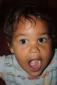# Can't replicate sem package in R

5 posts / 0 newOffline
Joined: 07/01/2010 - 03:02
Can't replicate sem package in R
AttachmentSizeSEMComputedR.pdf15.98 KBSEMComputedS.pdf16.13 KB

I'm slowly converting to OpenMX because of its fiml capabilities. I recently built two models that are shown in the enclosed attachments. You will notice that the correlation between T and P is identical in both models (whether S or D is used). However, when I try to replicate these results in OpenMX, I don't get identical results; they're usually about .04 different, which is quite significant for what I'm doing.

Here's my openMX code:

sem.model.S = mxModel("Two Factor Model Path Specification",
type="RAM",
dd,
manifestVars = names(data),
latentVars = c("T", "P"),
#### residual variances of observed
mxPath(
from=names(data)[-4],
arrows=2,
free=c(FALSE, FALSE, FALSE, TRUE, TRUE, TRUE, TRUE, TRUE, TRUE),
values=1,
labels=c("z1","z2","z3",paste("e", 1:6, sep=""))
),
# exogenous variances and covariance
mxPath(
from=c("S", "P", "T"),
arrows=2,
all=TRUE,
free=c(TRUE, TRUE, TRUE, TRUE, FALSE, TRUE, TRUE, TRUE, FALSE),
values=rep(1, times=9),
labels=c("varS", "cov1", "cov2",
"cov1", "varP", "cov3",
"cov2", "cov3", "varT")
),
mxPath(
from="T",
to=c("X1","X2","X3"),
arrows=1,
free=c(TRUE,TRUE,TRUE),
values=c(1,1,1),
labels=c("l1","l2","l3")
),
mxPath(
from="P",
to=c("Y1","Y2","Y3"),
arrows=1,
free=c(TRUE,TRUE,TRUE),
values=c(1,1,1),
labels=c("l4","l5","l6")
),
mxPath(
from=names(data)[1:3],
to=c("S"),
arrows=1,
free=TRUE,
values=c(1,1,1),
labels=c("z1l", "z2l", "z3l")
),

#means
mxPath(
from="one",
to=c("Interview", "Personality", "IQ", "X1","X2","X3","Y1","Y2","Y3","S","T","P"),
arrows=1,
free=c(rep(TRUE, times=10), FALSE, FALSE),
values=c(rep(1, times=10), 0, 0),
labels=c("meanInt", "meanPers", "meanIQ","meanx1","meanx2","meanx3",
"meany1","meany2","meany3","meanS",NA, NA)
)


)

The other model is nearly identical, except I replace "S" with "D"....could it be because I'm estimating means now when before I was using a correlation matrix? S and D are on different scales, but I wouldn't think it would matter that much.

Thanks!Offline
Joined: 07/31/2009 - 15:24
I don't have enough

I don't have enough experience to determine if the model in your script has been specified correctly. A general piece of advice I can provide is to specify lower bounds of 0 for your free parameters, if you want all your free parameters to have non-negative values. You can add the lbound = 0 argument to the invocations of the mxPath() function.Offline
Joined: 11/11/2011 - 22:18
Plan B: start small

Dustin, it would be nice if someone spots something specific in your model. If not, I think you should start small (like two variables and one path), and build up incrementally with both packages. I'll will take an hour, but that's the only way I'd feel comfortable with substantial discrepancies between approaches (no matter what software is involved).Offline
Joined: 07/31/2009 - 14:25
why do two different things differ?

you want us to compare two models... one of which you provide, the other you specify as "nearly" identical"

I'm not sure anyone could answer this question... why not provide the second model?

tOffline
Joined: 07/31/2009 - 15:26
Are you using the same

Are you using the same estimation method in the models fitted in the sem package and in OpenMx, or are you using the covariance-based ML method in the sem package and the raw data-based FIML method in OpenMx? Just the switch from ML to FIML could account for the difference in results.

Also, it appears that the model in your original post has no data. Is dd the mxData object? Is it raw data (type="raw") or covariance data (type="cov")?